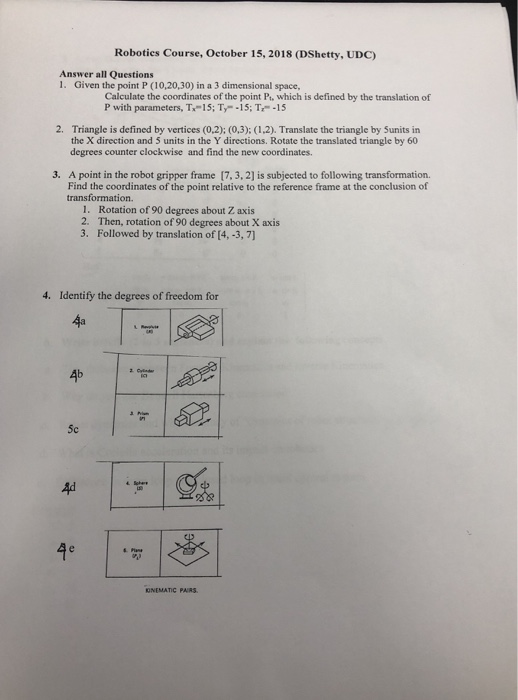# Question Robotics Course, October 15, 2018 (DShetty, UDC) Answer all Questions 1. Given the point P (10,20,30) in a 3 dimensional space, Calculate the coordinates of the point Pt, which is defined by the translation of P with parameters, T15; Ty-15; T-15 2. Triangle is defined by vertices (0,2); (0,3); (1.2). Translate the triangle by Sunits in the X direction and 5 Robotics Course, October 15, 2018 (DShetty, UDC) Answer all Questions 1. Given the point P (10,20,30) in a 3 dimensional space, Calculate the coordinates of the point Pt, which is defined by the translation of P with parameters, T15; Ty-15; T-15 2. Triangle is defined by vertices (0,2); (0,3); (1.2). Translate the triangle by Sunits in the X direction and 5 units in the Y directions. Rotate the translated triangle by 60 degrees counter clockwise and find the new coordinates. 3. A point in the robot gripper frame 7,3,2] is subjected to following transformation. Find the coordinates of the point relative to the reference frame at the conclusion of transformation. 1. Rotation of 90 degrees about Z axis Then, rotation of 90 degrees about X axis 2. 3. Followed by translation of 14,-3,7) 4. Identify the degrees of freedom for 4b ate 5c 4e CNEMATİC PARs.S74SNQ The Asker · Mechanical EngineeringTranscribed Image Text: Robotics Course, October 15, 2018 (DShetty, UDC) Answer all Questions 1. Given the point P (10,20,30) in a 3 dimensional space, Calculate the coordinates of the point Pt, which is defined by the translation of P with parameters, T15; Ty-15; T-15 2. Triangle is defined by vertices (0,2); (0,3); (1.2). Translate the triangle by Sunits in the X direction and 5 units in the Y directions. Rotate the translated triangle by 60 degrees counter clockwise and find the new coordinates. 3. A point in the robot gripper frame 7,3,2] is subjected to following transformation. Find the coordinates of the point relative to the reference frame at the conclusion of transformation. 1. Rotation of 90 degrees about Z axis Then, rotation of 90 degrees about X axis 2. 3. Followed by translation of 14,-3,7) 4. Identify the degrees of freedom for 4b ate 5c 4e CNEMATİC PARs.
More
Transcribed Image Text: Robotics Course, October 15, 2018 (DShetty, UDC) Answer all Questions 1. Given the point P (10,20,30) in a 3 dimensional space, Calculate the coordinates of the point Pt, which is defined by the translation of P with parameters, T15; Ty-15; T-15 2. Triangle is defined by vertices (0,2); (0,3); (1.2). Translate the triangle by Sunits in the X direction and 5 units in the Y directions. Rotate the translated triangle by 60 degrees counter clockwise and find the new coordinates. 3. A point in the robot gripper frame 7,3,2] is subjected to following transformation. Find the coordinates of the point relative to the reference frame at the conclusion of transformation. 1. Rotation of 90 degrees about Z axis Then, rotation of 90 degrees about X axis 2. 3. Followed by translation of 14,-3,7) 4. Identify the degrees of freedom for 4b ate 5c 4e CNEMATİC PARs.